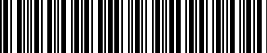## On the Subject of Barcode Cipher

I didn't realise we were going shopping.

• You are presented with 3 barcodes, a 6 digit number on a display, and an input display that cycles from the numbers 0 to 9.
• To solve the module, you must input a number for each barcode.
• Upon inputting a number for a barcode, both displays will hide their respective information.
• Upon receiving a strike, they will become visible again.
• Each barcode refers to a piece of edgework (refer to Table 1).
• Find the count of the edgework each barcode refers to (in the case of "Serial Number", use the sum of all the characters where A=1, B=2 etc.).
• To find the numbers for each barcode, you must first split the 6-digit number displayed into three 2-digit numbers.
• XOR the first 2-digit number with (the first barcode number × the sum of the digits of the 6-digit display). Repeat this step for the next 2-digit numbers and next barcode numbers.
• Modulo all of these numbers with (the last digit of the serial number % 5 + 5)
• Submit each number by changing the input display to the input number, then pressing the respective barcode.

### Table 1

Edgework Barcode
Serial Number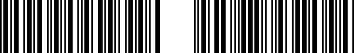Batteries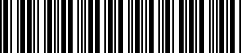Battery Holders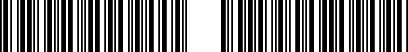Ports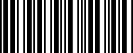Port Plates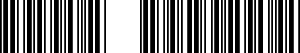Lit Indicators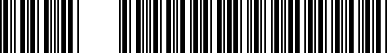Unlit Indicators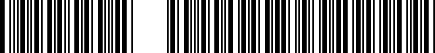Indicators